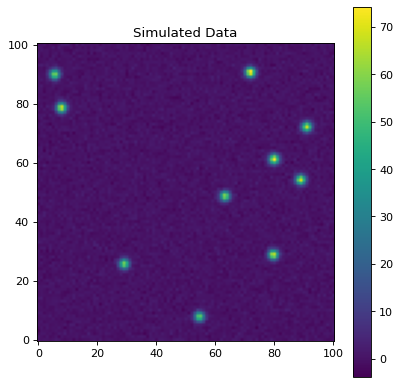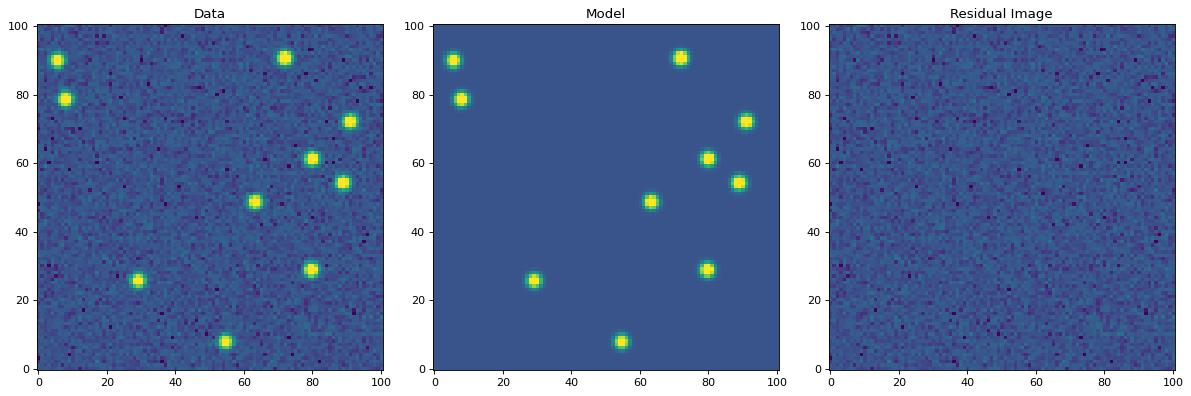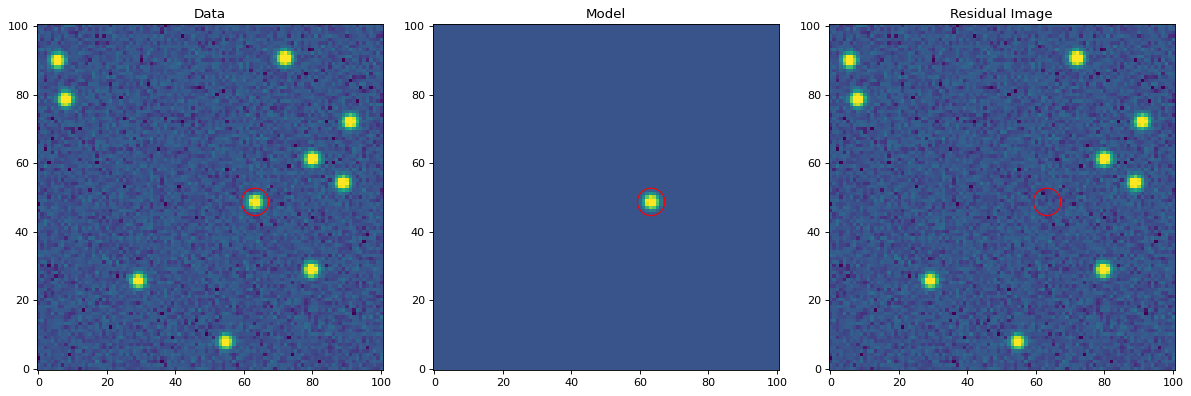PSF Photometry (photutils.psf)¶

The photutils.psf module contains tools for model-fitting photometry, often called “PSF photometry”.

Terminology¶

Different astronomy sub-fields use the terms “PSF”, “PRF”, or related terms somewhat differently, especially when colloquial usage is taken into account. This package aims to be at the very least internally consistent, following the definitions described here.

For this module we take Point Spread Function (PSF), or instrumental Point Spread Function (iPSF) to be the infinite resolution and infinite signal-to-noise flux distribution from a point source on the detector, after passing through optics, dust, atmosphere, etc. By contrast, the function describing the responsivity variations across individual pixels is the Pixel Response Function (sometimes called “PRF”, but that acronym is not used here for reasons that will soon be apparent). The convolution of the PSF and pixel response function, when discretized onto the detector (i.e., a rectilinear CCD grid), is the effective PSF (ePSF) or Point Response Function (PRF). (This latter terminology is the definition used by Spitzer. In many cases the PSF/ePSF/PRF distinction is unimportant, and the ePSF/PRF are simply called the “PSF”, but the distinction can be critical when dealing carefully with undersampled data or detectors with significant intra-pixel sensitivity variations. For a more detailed description of this formalism, see Anderson & King 2000.

All this said, in colloquial usage “PSF photometry” sometimes refers to the more general task of model-fitting photometry (with the effects of the PSF either implicitly or explicitly included in the models), regardless of exactly what kind of model is actually being fit. For brevity (e.g., photutils.psf), we use “PSF photometry” in this way, as a shorthand for the general approach.

Building an effective PSF (ePSF)¶

Please see Building an effective Point Spread Function (ePSF) for documentation on how to build an ePSF.

PSF Photometry¶

Photutils provides a modular set of tools to perform PSF photometry for different science cases. These are implemented as separate classes to do sub-tasks of PSF photometry. It also provides high-level classes that connect these pieces together. In particular, it contains an implementation of the DAOPHOT algorithm (DAOPhotPSFPhotometry) proposed by Stetson in his seminal paper for crowded-field stellar photometry.

The DAOPHOT algorithm consists in applying the loop FIND, GROUP, NSTAR, SUBTRACT, FIND until no more stars are detected or a given number of iterations is reached. Basically, DAOPhotPSFPhotometry works as follows. The first step is to estimate the sky background. For this task, photutils provides several classes to compute scalar and 2D backgrounds, see background for details. The next step is to find an initial estimate of the positions of potential sources. This can be accomplished by using source detection algorithms, which are implemented in detection.

After finding sources, one would apply a clustering algorithm in order to label the sources according to groups. Usually, those groups are formed by a distance criterion, which is the case of the grouping algorithm proposed by Stetson. In DAOGroup, we provide an implementation of that algorithm. In addition, DBSCANGroup can also be used to group sources with more complex distance criteria. The reason behind the construction of groups is illustrated as follows: imagine that one would like to fit 300 stars and the model for each star has three parameters to be fitted. If one constructs a single model to fit the 300 stars simultaneously, then the optimization algorithm will have to search for the solution in a 900 dimensional space, which is computationally expensive and error-prone. Reducing the stars in groups effectively reduces the dimension of the parameter space, which facilitates the optimization process.

Provided that the groups are available, the next step is to fit the sources simultaneously for each group. This task can be done using an astropy fitter, for instance, LevMarLSQFitter.

After sources are fitted, they are subtracted from the given image and, after fitting all sources, the residual image is analyzed by the finding routine again in order to check if there exist any source which has not been detected previously. This process goes on until no more sources are identified by the finding routine.

Note

It is important to note the conventions on the column names of the input/output astropy Tables which are passed along to the source detection and photometry objects. For instance, all source detection objects should output a table with columns named as xcentroid and ycentroid (check detection). On the other hand, DAOGroup expects columns named as x_0 and y_0, which represents the initial guesses on the sources’ centroids. Finally, the output of the fitting process shows columns named as x_fit, y_fit, flux_fit for the optimum values and x_0, y_0, flux_0 for the initial guesses. Although this convention implies that the columns have to be renamed along the process, it has the advantage of clarity so that one can keep track and easily differentiate where input/outputs came from.

High-Level Structure¶

Photutils provides three classes to perform PSF Photometry: BasicPSFPhotometry, IterativelySubtractedPSFPhotometry, and DAOPhotPSFPhotometry. Together these provide the core workflow to make photometric measurements given an appropriate PSF (or other) model.

BasicPSFPhotometry implements the minimum tools for model-fitting photometry. At its core, this involves finding sources in an image, grouping overlapping sources into a single model, fitting the model to the sources, and subtracting the models from the image. In DAOPHOT parlance, this is essentially running the “FIND, GROUP, NSTAR, SUBTRACT” once. Because it is only a single cycle of that sequence, this class should be used when the degree of crowdedness of the field is not very high, for instance, when most stars are separated by a distance no less than one FWHM and their brightness are relatively uniform. It is critical to understand, though, that BasicPSFPhotometry does not actually contain the functionality to do all these steps - that is provided by other objects (or can be user-written) functions. Rather, it provides the framework and data structures in which these operations run. Because of this, BasicPSFPhotometry is particularly useful for build more complex workflows, as all the stages can be turned on or off or replaced with different implementations as the user desires.

IterativelySubtractedPSFPhotometry is similar to BasicPSFPhotometry, but it adds a parameter called n_iters which is the number of iterations for which the loop “FIND, GROUP, NSTAR, SUBTRACT, FIND…” will be performed. This class enables photometry in a scenario where there exists significant overlap between stars that are of quite different brightness. For instance, the detection algorithm may not be able to detect a faint and bright star very close together in the first iteration, but they will be detected in the next iteration after the brighter stars have been fit and subtracted. Like BasicPSFPhotometry, it does not include implementations of the stages of this process, but it provides the structure in which those stages run.

DAOPhotPSFPhotometry is a special case of IterativelySubtractedPSFPhotometry. Unlike IterativelySubtractedPSFPhotometry and BasicPSFPhotometry, the class includes specific implementations of the stages of the photometric measurements, tuned to reproduce the algorithms used for the DAOPHOT code. Specifically, the finder, group_maker, bkg_estimator attributes are set to the DAOStarFinder, DAOGroup, and MMMBackground, respectively. Therefore, users need to input the parameters of those classes to set up a DAOPhotPSFPhotometry object, rather than providing objects to do these stages (which is what the other classes require).

Those classes and all the classes they use for the steps in the photometry process can always be replaced by user-supplied functions if you wish to customize any stage of the photometry process. This makes the machinery very flexible, while still providing a “batteries included” approach with a default implementation that’s suitable for many use cases.

Basic Usage¶

The basic usage of, e.g., IterativelySubtractedPSFPhotometry is as follows:

>>> # create an IterativelySubtractedPSFPhotometry object
>>> from photutils.psf import IterativelySubtractedPSFPhotometry
>>> my_photometry = IterativelySubtractedPSFPhotometry(
...     finder=my_finder, group_maker=my_group_maker,
...     bkg_estimator=my_bkg_estimator, psf_model=my_psf_model,
...     fitter=my_fitter, niters=3, fitshape=(7, 7))
>>> # get photometry results
>>> photometry_results = my_photometry(image=my_image)
>>> # get residual image
>>> residual_image = my_photometry.get_residual_image()

Where my_finder, my_group_maker, and my_bkg_estimator may be any suitable class or callable function. This approach allows one to customize every part of the photometry process provided that their input/output are compatible with the input/output expected by IterativelySubtractedPSFPhotometry. photutils.psf provides all the necessary classes to reproduce the DAOPHOT algorithm, but any individual part of that algorithm can be swapped for a user-defined function. See the API documentation for precise details on what these classes or functions should look like.

Performing PSF Photometry¶

Let’s take a look at a simple example with simulated stars whose PSF is assumed to be Gaussian.

First let’s create an image with four overlapping stars:

>>> import numpy as np
>>> from astropy.table import Table
>>> from photutils.datasets import (make_noise_image,
...                                 make_gaussian_sources_image)
>>> sigma_psf = 2.0
>>> sources = Table()
>>> sources['flux'] = [700, 800, 700, 800]
>>> sources['x_mean'] = [12, 17, 12, 17]
>>> sources['y_mean'] = [15, 15, 20, 20]
>>> sources['x_stddev'] = sigma_psf * np.ones(4)
>>> sources['y_stddev'] = sources['x_stddev']
>>> sources['theta'] = [0, 0, 0, 0]
>>> sources['id'] = [1, 2, 3, 4]
>>> tshape = (32, 32)
>>> image = (make_gaussian_sources_image(tshape, sources) +
...          make_noise_image(tshape, distribution='poisson', mean=6.,
...                           seed=123) +
...          make_noise_image(tshape, distribution='gaussian', mean=0.,
...                           stddev=2., seed=123))
>>> from matplotlib import rcParams
>>> rcParams['font.size'] = 13
>>> import matplotlib.pyplot as plt
>>> plt.imshow(image, cmap='viridis', aspect=1, interpolation='nearest',
...            origin='lower')
>>> plt.title('Simulated data')Then let’s import the required classes to set up a IterativelySubtractedPSFPhotometry object:

>>> from photutils.detection import IRAFStarFinder
>>> from photutils.psf import IntegratedGaussianPRF, DAOGroup
>>> from photutils.background import MMMBackground, MADStdBackgroundRMS
>>> from astropy.modeling.fitting import LevMarLSQFitter
>>> from astropy.stats import gaussian_sigma_to_fwhm

Let’s then instantiate and use the objects:

>>> std = bkgrms(image)
>>> iraffind = IRAFStarFinder(threshold=3.5*std,
...                           fwhm=sigma_psf * gaussian_sigma_to_fwhm,
...                           minsep_fwhm=0.01, roundhi=5.0, roundlo=-5.0,
...                           sharplo=0.0, sharphi=2.0)
>>> daogroup = DAOGroup(2.0 * sigma_psf * gaussian_sigma_to_fwhm)
>>> mmm_bkg = MMMBackground()
>>> fitter = LevMarLSQFitter()
>>> psf_model = IntegratedGaussianPRF(sigma=sigma_psf)
>>> from photutils.psf import IterativelySubtractedPSFPhotometry
>>> photometry = IterativelySubtractedPSFPhotometry(finder=iraffind,
...                                                 group_maker=daogroup,
...                                                 bkg_estimator=mmm_bkg,
...                                                 psf_model=psf_model,
...                                                 fitter=LevMarLSQFitter(),
...                                                 niters=1, fitshape=(11, 11))
>>> result_tab = photometry(image=image)
>>> residual_image = photometry.get_residual_image()

Note that the parameters values for the finder class, i.e., IRAFStarFinder, are completely chosen in an arbitrary manner and optimum values do vary according to the data.

As mentioned before, the way to actually do the photometry is by using photometry as a function-like call.

It’s worth noting that image does not need to be background subtracted. The subtraction is done during the photometry process with the attribute bkg that was used to set up photometry.

Now, let’s compare the simulated and the residual images:

>>> plt.subplot(1, 2, 1)
>>> plt.imshow(image, cmap='viridis', aspect=1, interpolation='nearest',
origin='lower')
>>> plt.title('Simulated data')
>>> plt.subplot(1, 2, 2)
>>> plt.imshow(residual_image, cmap='viridis', aspect=1,
...            interpolation='nearest', origin='lower')
>>> plt.title('Residual Image')
>>> plt.show()Performing PSF Photometry with Fixed Centroids¶

In case that the centroids positions of the stars are known a priori, then they can be held fixed during the fitting process and the optimizer will only consider flux as a variable. To do that, one has to set the fixed attribute for the centroid parameters in psf as True.

Consider the previous example after the line psf_model = IntegratedGaussianPRF(sigma=sigma_psf):

>>> psf_model.x_0.fixed = True
>>> psf_model.y_0.fixed = True
>>> pos = Table(names=['x_0', 'y_0'], data=[sources['x_mean'],
...                                         sources['y_mean']])
>>> photometry = BasicPSFPhotometry(group_maker=daogroup,
...                                 bkg_estimator=mmm_bkg,
...                                 psf_model=psf_model,
...                                 fitter=LevMarLSQFitter(),
...                                 fitshape=(11, 11))
>>> result_tab = photometry(image=image, init_guesses=pos)
>>> residual_image = photometry.get_residual_image()
>>> plt.subplot(1, 2, 1)
>>> plt.imshow(image, cmap='viridis', aspect=1,
...            interpolation='nearest', origin='lower')
>>> plt.title('Simulated data')
>>> plt.subplot(1, 2, 2)
>>> plt.imshow(residual_image, cmap='viridis', aspect=1,
...            interpolation='nearest', origin='lower')
>>> plt.title('Residual Image')The PSF photometry classes can also be used to fit more model parameters than just the flux and center positions. While a more realistic use case might be fitting sky backgrounds, or shape parameters of galaxies, here we use the sigma parameter in IntegratedGaussianPRF as the simplest possible example of this feature. (For actual PSF photometry of stars you would not want to do this, because the shape of the PSF should be set by bright stars or an optical model and held fixed when fitting.)

First, let us instantiate a PSF model object:

>>> gaussian_prf = IntegratedGaussianPRF()

The attribute fixed for the sigma parameter is set to True by default, i.e., sigma is not considered during the fitting process. Let’s first change this behavior:

>>> gaussian_prf.sigma.fixed = False

In addition, we need to indicate the initial guess which will be used in during the fitting process. By the default, the initial guess is taken as the default value of sigma, but we can change that by doing:

>>> gaussian_prf.sigma.value = 2.05

Now, let’s create a simulated image which has a brighter star and one overlapping fainter companion so that the detection algorithm won’t be able to identify it, and hence we should use IterativelySubtractedPSFPhotometry to measure the fainter star as well. Also, note that both of the stars have sigma=2.0.

import numpy as np
import matplotlib.pyplot as plt
from matplotlib.colors import LogNorm
from photutils.datasets import (make_noise_image,
make_gaussian_sources_image)
from astropy.table import Table

sources = Table()
sources['flux'] = [10000, 1000]
sources['x_mean'] = [18, 9]
sources['y_mean'] = [17, 21]
sources['x_stddev'] =  * 2
sources['y_stddev'] = sources['x_stddev']
sources['theta'] =  * 2
tshape = (32, 32)
image = (make_gaussian_sources_image(tshape, sources)
+ make_noise_image(tshape, distribution='poisson', mean=6.,
seed=123)
+ make_noise_image(tshape, distribution='gaussian', mean=0.,
stddev=2., seed=123))

vmin, vmax = np.percentile(image, [5, 95])
plt.imshow(image, cmap='viridis', aspect=1, interpolation='nearest',
origin='lower', norm=LogNorm(vmin=vmin, vmax=vmax))Let’s instantiate the necessary objects in order to use an IterativelySubtractedPSFPhotometry to perform photometry:

>>> daogroup = DAOGroup(crit_separation=8)
>>> mmm_bkg = MMMBackground()
>>> iraffind = IRAFStarFinder(threshold=2.5 * mmm_bkg(image), fwhm=4.5)
>>> fitter = LevMarLSQFitter()
>>> gaussian_prf = IntegratedGaussianPRF(sigma=2.05)
>>> gaussian_prf.sigma.fixed = False
>>> itr_phot_obj = IterativelySubtractedPSFPhotometry(finder=iraffind,
...                                                   group_maker=daogroup,
...                                                   bkg_estimator=mmm_bkg,
...                                                   psf_model=gaussian_prf,
...                                                   fitter=fitter,
...                                                   fitshape=(11, 11),
...                                                   niters=2)

Now, let’s use the callable itr_phot_obj to perform photometry:

>>> phot_results = itr_phot_obj(image)
>>> phot_results['id', 'group_id', 'iter_detected', 'x_0', 'y_0', 'flux_0']
id group_id iter_detected      x_0           y_0          flux_0
--- -------- ------------- ------------- ------------- -------------
1        1             1 18.0045935148 17.0060558543 9437.07321281
1        1             2 9.06141447183 21.0680052846 977.163727416
>>> phot_results['sigma_0', 'sigma_fit', 'x_fit', 'y_fit', 'flux_fit']
sigma_0   sigma_fit       x_fit         y_fit        flux_fit
------- ------------- ------------- ------------- -------------
2.05 1.98092026939 17.9995106906 17.0039419384 10016.4470148
2.05 1.98516037471 9.12116345703 21.0599164498 1036.79115883

We can see that sigma_0 (the initial guess for sigma) was assigned to the value we used when creating the PSF model.

Let’s take a look at the residual image:

>>> plt.imshow(itr_phot_obj.get_residual_image(), cmap='viridis',
... aspect=1, interpolation='nearest', origin='lower')Reference/API¶

This subpackage contains tools to perform point-spread-function (PSF) photometry.

Functions¶

 create_matching_kernel(source_psf, target_psf) Create a kernel to match 2D point spread functions (PSF) using the ratio of Fourier transforms. extract_stars(data, catalogs[, size]) Extract cutout images centered on stars defined in the input catalog(s). get_grouped_psf_model(template_psf_model, …) Construct a joint PSF model which consists of a sum of PSF’s templated on a specific model, but whose parameters are given by a table of objects. prepare_psf_model(psfmodel[, xname, yname, …]) Convert a 2D PSF model to one suitable for use with BasicPSFPhotometry or its subclasses. resize_psf(psf, input_pixel_scale, …[, order]) Resize a PSF using spline interpolation of the requested order. subtract_psf(data, psf, posflux[, subshape]) Subtract PSF/PRFs from an image.

Classes¶

 BasicPSFPhotometry(group_maker, …[, …]) This class implements a PSF photometry algorithm that can find sources in an image, group overlapping sources into a single model, fit the model to the sources, and subtracting the models from the image. CosineBellWindow(alpha) Class to define a 2D cosine bell window function. DAOGroup(crit_separation) This class implements the DAOGROUP algorithm presented by Stetson (1987). DAOPhotPSFPhotometry(crit_separation, …[, …]) This class implements an iterative algorithm based on the DAOPHOT algorithm presented by Stetson (1987) to perform point spread function photometry in crowded fields. DBSCANGroup(crit_separation[, min_samples, …]) Class to create star groups according to a distance criteria using the Density-based Spatial Clustering of Applications with Noise (DBSCAN) from scikit-learn. EPSFBuilder([oversampling, shape, …]) Class to build an effective PSF (ePSF). EPSFFitter([fitter, fit_boxsize]) Class to fit an ePSF model to one or more stars. EPSFModel(data[, flux, x_0, y_0, normalize, …]) A class that models an effective PSF (ePSF). EPSFStar(data[, weights, cutout_center, …]) A class to hold a 2D cutout image and associated metadata of a star used to build an ePSF. EPSFStars(stars_list) Class to hold a list of EPSFStar and/or LinkedEPSFStar objects. FittableImageModel(data[, flux, x_0, y_0, …]) A fittable 2D model of an image allowing for image intensity scaling and image translations. GriddedPSFModel(data[, flux, x_0, y_0, …]) A fittable 2D model containing a grid PSF models defined at specific locations that are interpolated to evaluate a PSF at an arbitrary (x, y) position. This base class provides the basic interface for subclasses that are capable of classifying stars in groups. Class to define a 2D Hanning (or Hann) window function. IntegratedGaussianPRF([sigma, x_0, y_0, flux]) Circular Gaussian model integrated over pixels. This class implements an iterative algorithm to perform point spread function photometry in crowded fields. LinkedEPSFStar(stars_list) A class to hold a list of EPSFStar objects for linked stars. NonNormalizable Used to indicate that a FittableImageModel model is non-normalizable. PRFAdapter(psfmodel[, renormalize_psf, …]) A model that adapts a supplied PSF model to act as a PRF. SplitCosineBellWindow(alpha, beta) Class to define a 2D split cosine bell taper function. TopHatWindow(beta) Class to define a 2D top hat window function. TukeyWindow(alpha) Class to define a 2D Tukey window function.

photutils.psf.sandbox Module¶

This module stores work related to photutils.psf that is not quite ready for prime-time (i.e., is not considered a stable public API), but is included either for experimentation or as legacy code.

Classes¶

 DiscretePRF(prf_array[, normalize, subsampling]) A discrete Pixel Response Function (PRF) model. Reproject(wcs_original, wcs_rectified) Class to reproject pixel coordinates between unrectified and rectified images.